Скачать презентацию Ch 8 Profit Max Under Perfect Competition

2f938a3ced8654d0258b332a15e203a0.ppt

• Количество слайдов: 21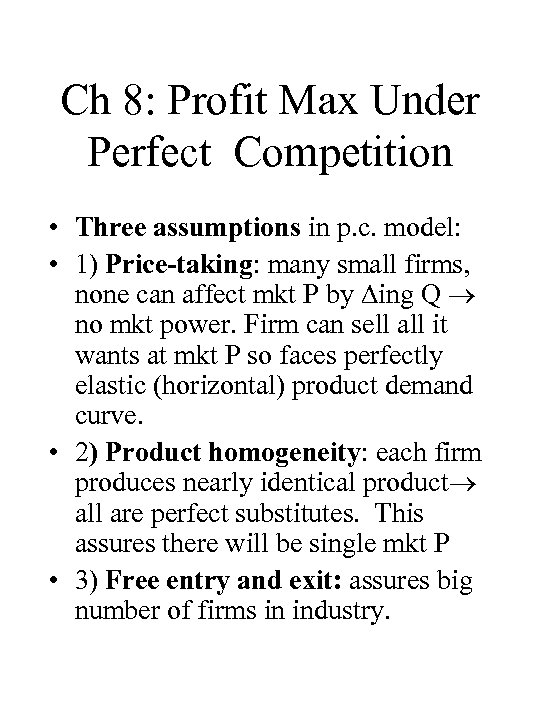Ch 8: Profit Max Under Perfect Competition • Three assumptions in p. c. model: • 1) Price-taking: many small firms, none can affect mkt P by ing Q no mkt power. Firm can sell all it wants at mkt P so faces perfectly elastic (horizontal) product demand curve. • 2) Product homogeneity: each firm produces nearly identical product all are perfect substitutes. This assures there will be single mkt P • 3) Free entry and exit: assures big number of firms in industry.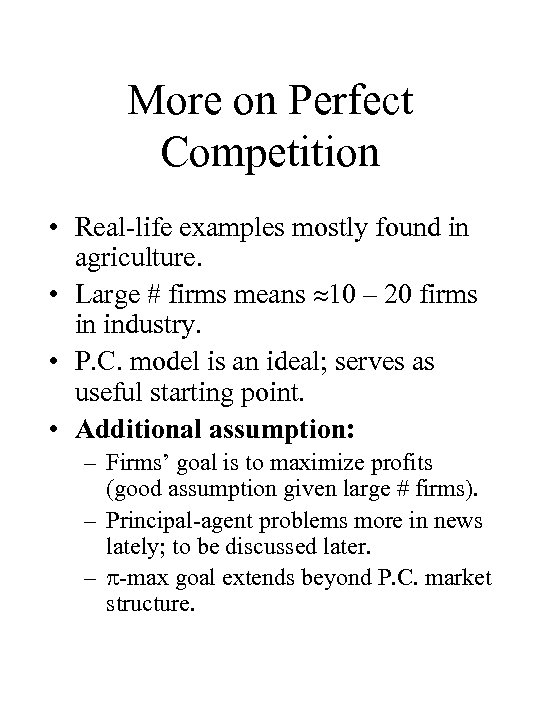More on Perfect Competition • Real-life examples mostly found in agriculture. • Large # firms means 10 – 20 firms in industry. • P. C. model is an ideal; serves as useful starting point. • Additional assumption: – Firms’ goal is to maximize profits (good assumption given large # firms). – Principal-agent problems more in news lately; to be discussed later. – -max goal extends beyond P. C. market structure.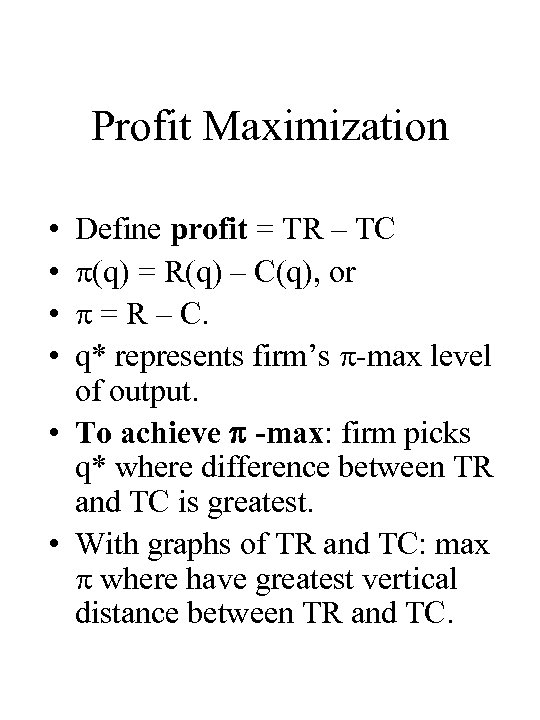Profit Maximization • • Define profit = TR – TC (q) = R(q) – C(q), or = R – C. q* represents firm’s -max level of output. • To achieve -max: firm picks q* where difference between TR and TC is greatest. • With graphs of TR and TC: max where have greatest vertical distance between TR and TC.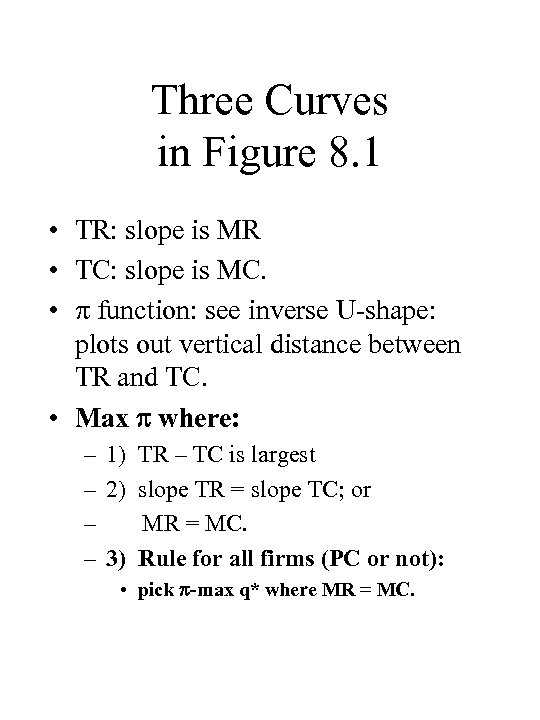Three Curves in Figure 8. 1 • TR: slope is MR • TC: slope is MC. • function: see inverse U-shape: plots out vertical distance between TR and TC. • Max where: – 1) TR – TC is largest – 2) slope TR = slope TC; or – MR = MC. – 3) Rule for all firms (PC or not): • pick -max q* where MR = MC.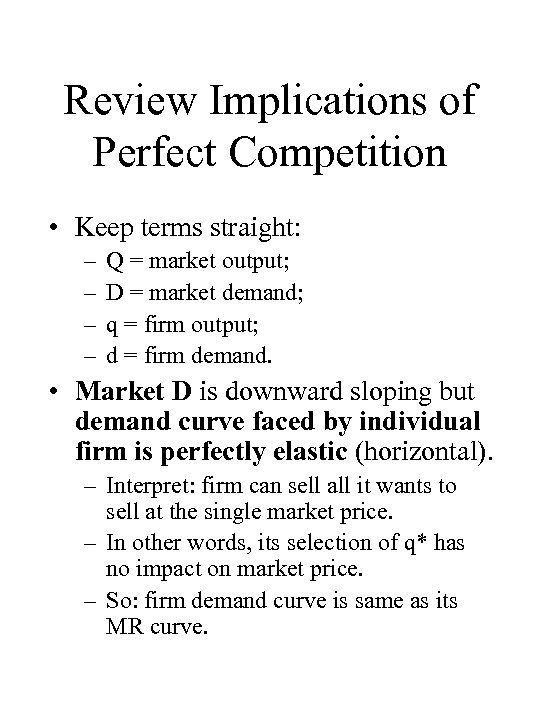Review Implications of Perfect Competition • Keep terms straight: – – Q = market output; D = market demand; q = firm output; d = firm demand. • Market D is downward sloping but demand curve faced by individual firm is perfectly elastic (horizontal). – Interpret: firm can sell all it wants to sell at the single market price. – In other words, its selection of q* has no impact on market price. – So: firm demand curve is same as its MR curve.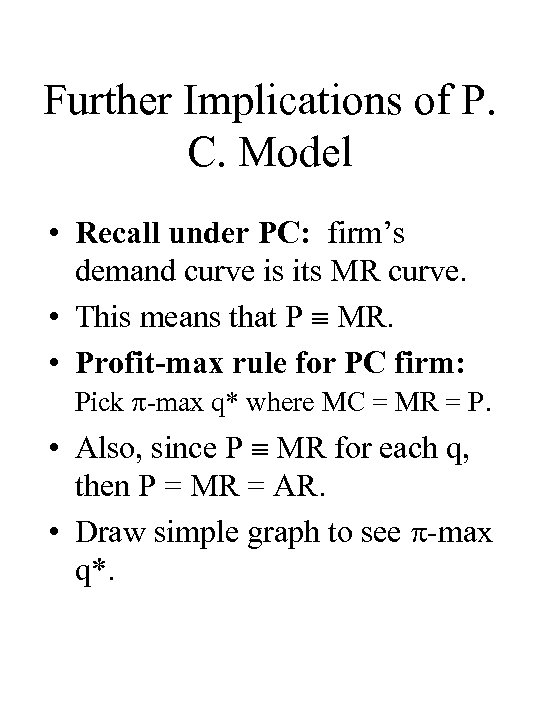Further Implications of P. C. Model • Recall under PC: firm’s demand curve is its MR curve. • This means that P MR. • Profit-max rule for PC firm: Pick -max q* where MC = MR = P. • Also, since P MR for each q, then P = MR = AR. • Draw simple graph to see -max q*.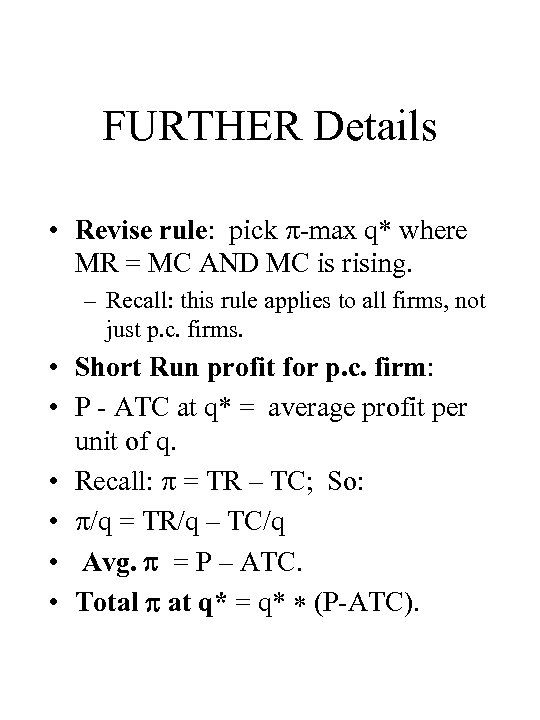FURTHER Details • Revise rule: pick -max q* where MR = MC AND MC is rising. – Recall: this rule applies to all firms, not just p. c. firms. • Short Run profit for p. c. firm: • P - ATC at q* = average profit per unit of q. • Recall: = TR – TC; So: • /q = TR/q – TC/q • Avg. = P – ATC. • Total at q* = q* (P-ATC).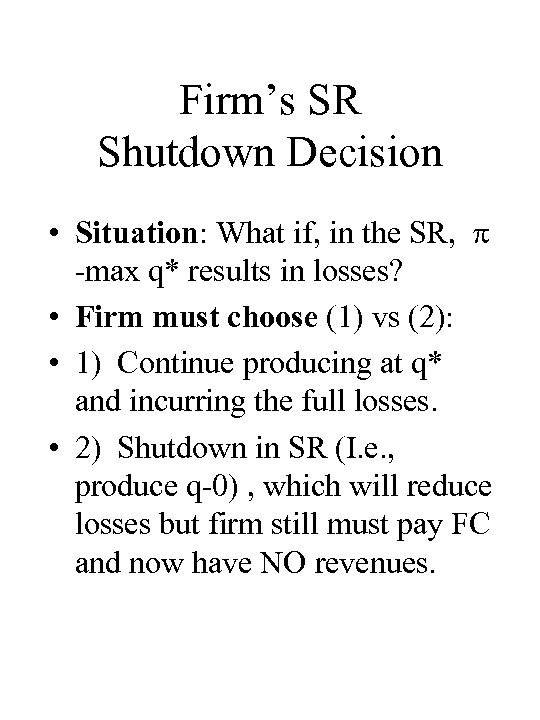Firm’s SR Shutdown Decision • Situation: What if, in the SR, -max q* results in losses? • Firm must choose (1) vs (2): • 1) Continue producing at q* and incurring the full losses. • 2) Shutdown in SR (I. e. , produce q-0) , which will reduce losses but firm still must pay FC and now have NO revenues.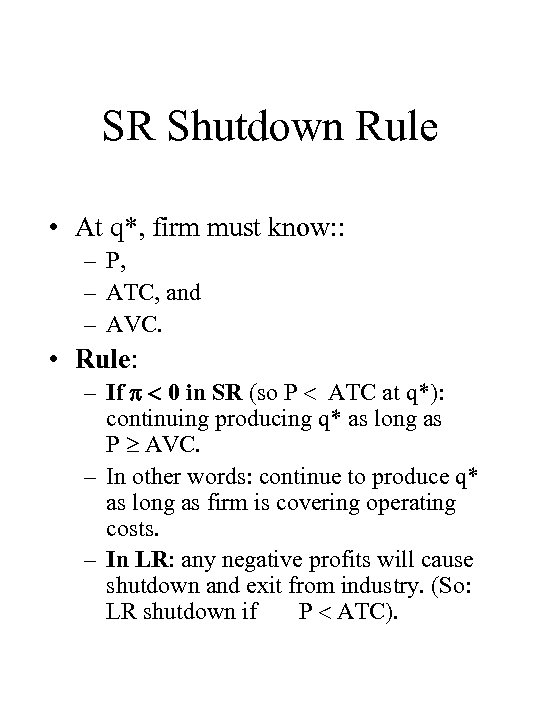SR Shutdown Rule • At q*, firm must know: : – P, – ATC, and – AVC. • Rule: – If 0 in SR (so P ATC at q*): continuing producing q* as long as P AVC. – In other words: continue to produce q* as long as firm is covering operating costs. – In LR: any negative profits will cause shutdown and exit from industry. (So: LR shutdown if P ATC).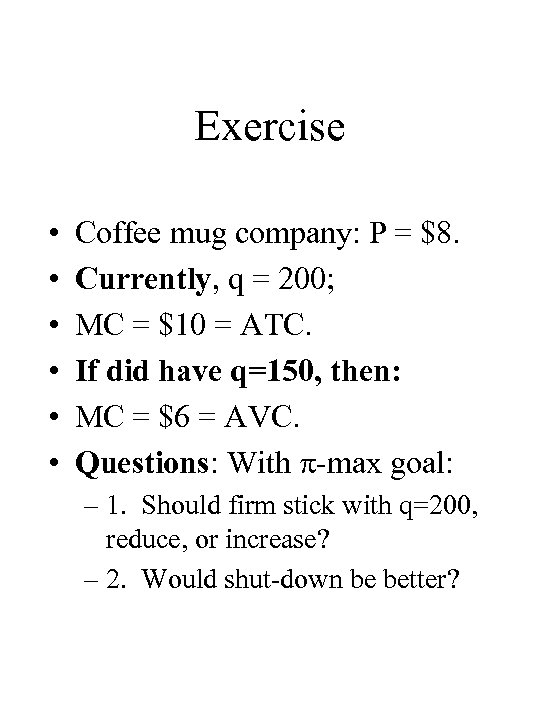Exercise • • • Coffee mug company: P = \$8. Currently, q = 200; MC = \$10 = ATC. If did have q=150, then: MC = \$6 = AVC. Questions: With -max goal: – 1. Should firm stick with q=200, reduce, or increase? – 2. Would shut-down be better?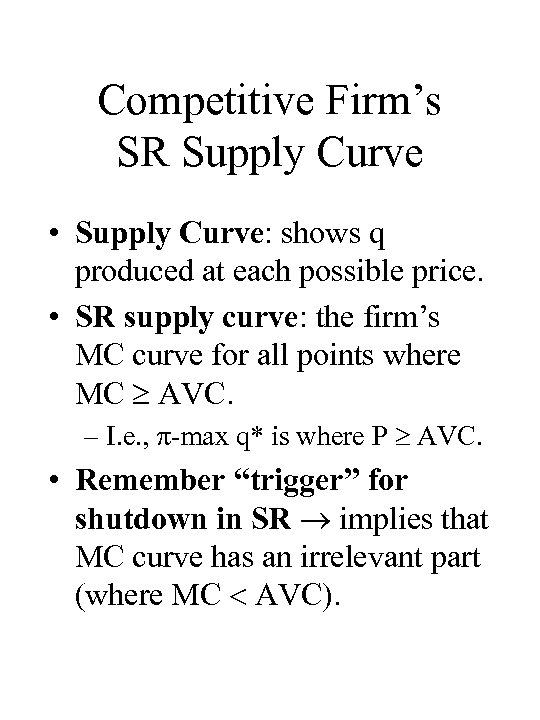Competitive Firm’s SR Supply Curve • Supply Curve: shows q produced at each possible price. • SR supply curve: the firm’s MC curve for all points where MC AVC. – I. e. , -max q* is where P AVC. • Remember “trigger” for shutdown in SR implies that MC curve has an irrelevant part (where MC AVC).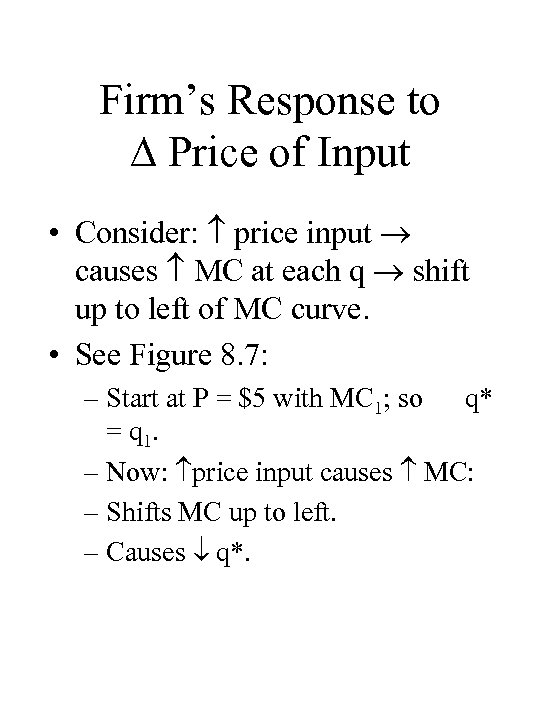Firm’s Response to Price of Input • Consider: price input causes MC at each q shift up to left of MC curve. • See Figure 8. 7: – Start at P = \$5 with MC 1; so q* = q 1. – Now: price input causes MC: – Shifts MC up to left. – Causes q*.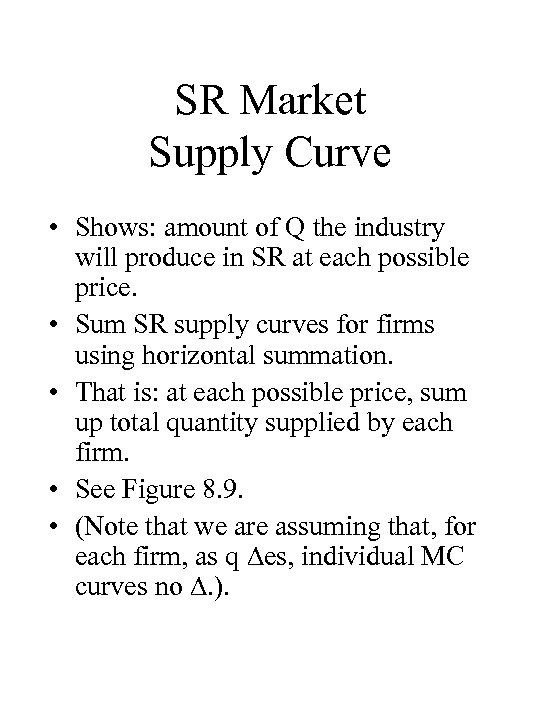SR Market Supply Curve • Shows: amount of Q the industry will produce in SR at each possible price. • Sum SR supply curves for firms using horizontal summation. • That is: at each possible price, sum up total quantity supplied by each firm. • See Figure 8. 9. • (Note that we are assuming that, for each firm, as q es, individual MC curves no . ).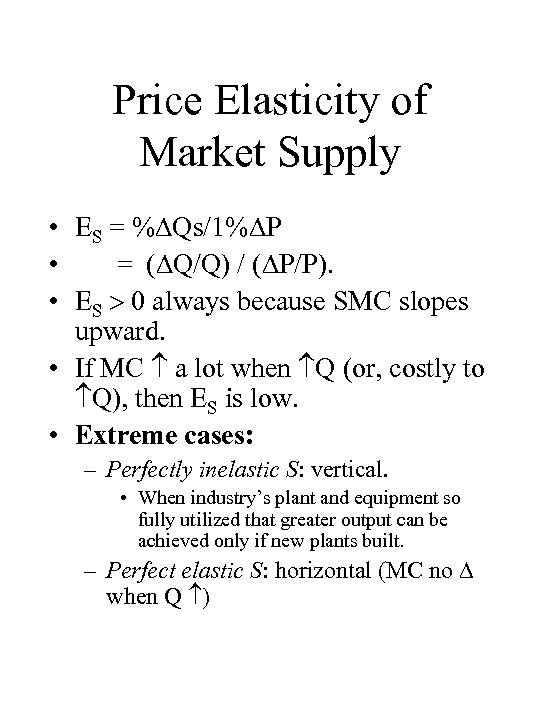Price Elasticity of Market Supply • ES = % Qs/1% P • = ( Q/Q) / ( P/P). • ES 0 always because SMC slopes upward. • If MC a lot when Q (or, costly to Q), then ES is low. • Extreme cases: – Perfectly inelastic S: vertical. • When industry’s plant and equipment so fully utilized that greater output can be achieved only if new plants built. – Perfect elastic S: horizontal (MC no when Q )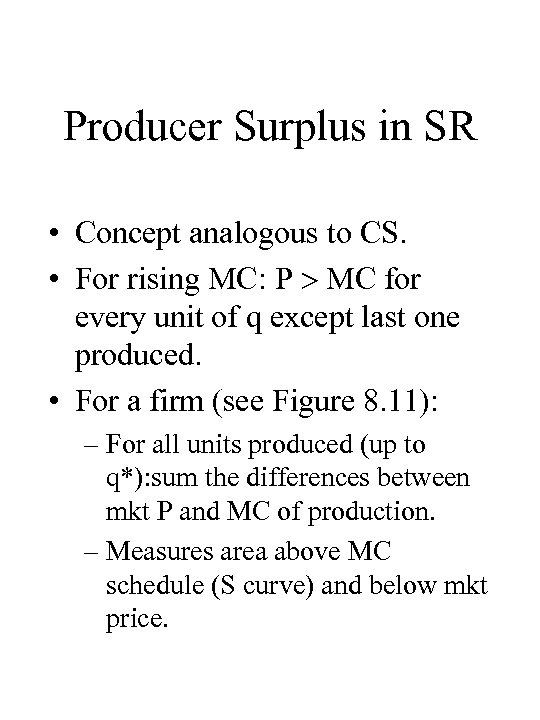Producer Surplus in SR • Concept analogous to CS. • For rising MC: P MC for every unit of q except last one produced. • For a firm (see Figure 8. 11): – For all units produced (up to q*): sum the differences between mkt P and MC of production. – Measures area above MC schedule (S curve) and below mkt price.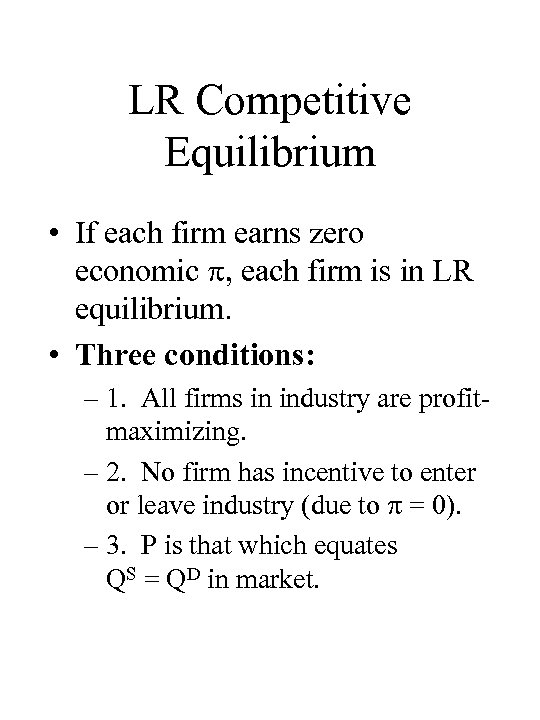LR Competitive Equilibrium • If each firm earns zero economic , each firm is in LR equilibrium. • Three conditions: – 1. All firms in industry are profitmaximizing. – 2. No firm has incentive to enter or leave industry (due to = 0). – 3. P is that which equates QS = QD in market.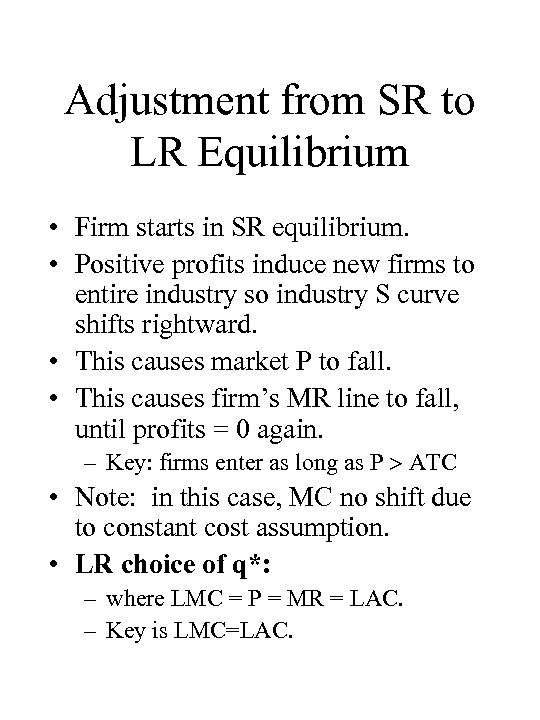Adjustment from SR to LR Equilibrium • Firm starts in SR equilibrium. • Positive profits induce new firms to entire industry so industry S curve shifts rightward. • This causes market P to fall. • This causes firm’s MR line to fall, until profits = 0 again. – Key: firms enter as long as P ATC • Note: in this case, MC no shift due to constant cost assumption. • LR choice of q*: – where LMC = P = MR = LAC. – Key is LMC=LAC.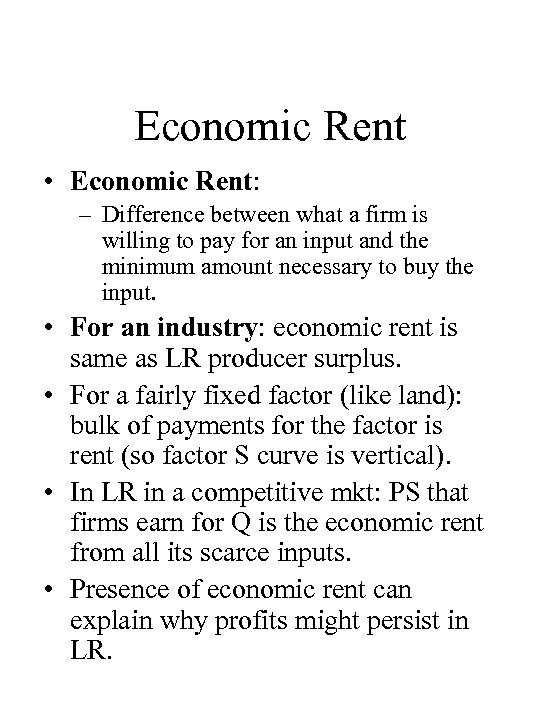Economic Rent • Economic Rent: – Difference between what a firm is willing to pay for an input and the minimum amount necessary to buy the input. • For an industry: economic rent is same as LR producer surplus. • For a fairly fixed factor (like land): bulk of payments for the factor is rent (so factor S curve is vertical). • In LR in a competitive mkt: PS that firms earn for Q is the economic rent from all its scarce inputs. • Presence of economic rent can explain why profits might persist in LR.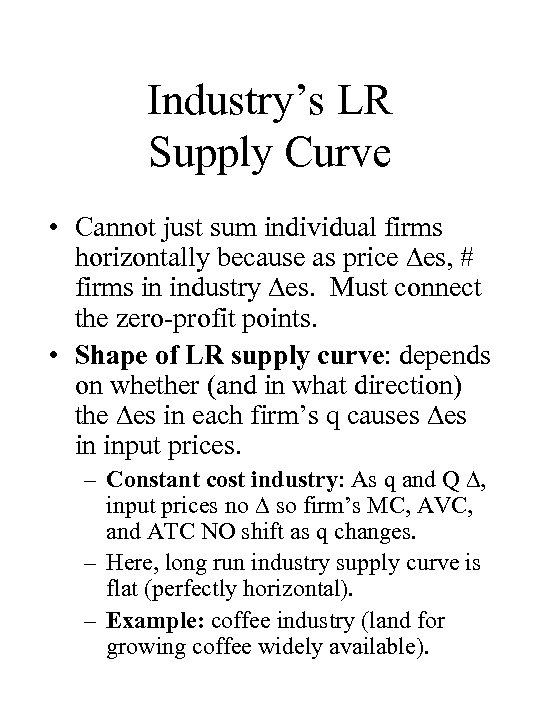Industry’s LR Supply Curve • Cannot just sum individual firms horizontally because as price es, # firms in industry es. Must connect the zero-profit points. • Shape of LR supply curve: depends on whether (and in what direction) the es in each firm’s q causes es in input prices. – Constant cost industry: As q and Q , input prices no so firm’s MC, AVC, and ATC NO shift as q changes. – Here, long run industry supply curve is flat (perfectly horizontal). – Example: coffee industry (land for growing coffee widely available).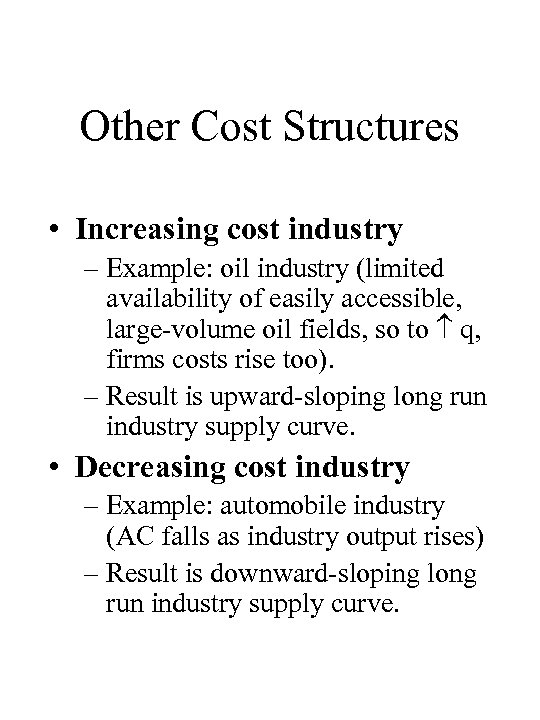Other Cost Structures • Increasing cost industry – Example: oil industry (limited availability of easily accessible, large-volume oil fields, so to q, firms costs rise too). – Result is upward-sloping long run industry supply curve. • Decreasing cost industry – Example: automobile industry (AC falls as industry output rises) – Result is downward-sloping long run industry supply curve.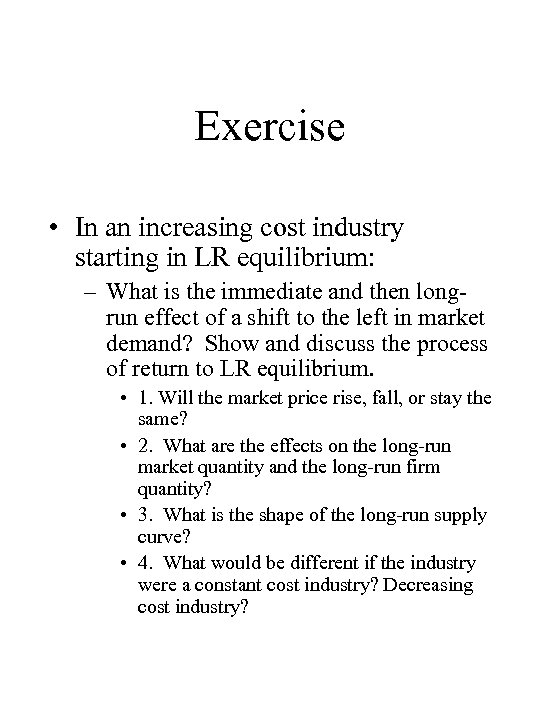Exercise • In an increasing cost industry starting in LR equilibrium: – What is the immediate and then longrun effect of a shift to the left in market demand? Show and discuss the process of return to LR equilibrium. • 1. Will the market price rise, fall, or stay the same? • 2. What are the effects on the long-run market quantity and the long-run firm quantity? • 3. What is the shape of the long-run supply curve? • 4. What would be different if the industry were a constant cost industry? Decreasing cost industry?